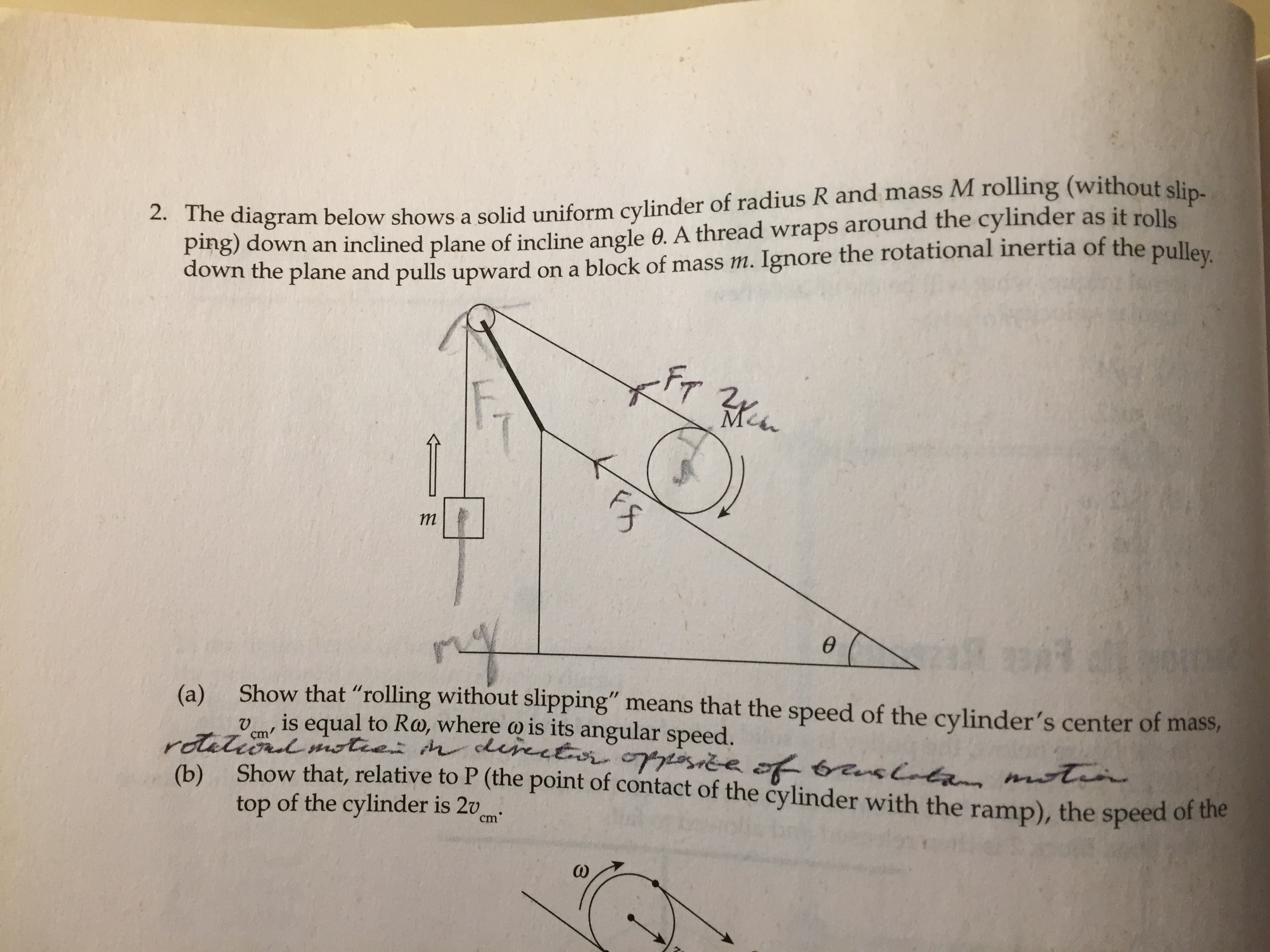## Proof of fundamental theorem of arithmetic

I read it a couple days ago and actually remembered it this time in a way that I will never forget it. It invokes Euclid’s lemma, which states that if$p | ab$ for$p$ prime, then$p | a$ or$p | b$, which can be proved using Bezout’s lemma. For existence, it does induction on the number of factors, with$1$ as the trivial base case. For the non base case, wherein our number is composite, apply the inductive hypothesis on the factors. For uniqueness, assume two distinct factorizations:$p_1p_2\ldots p_n = q_1q_2\ldots q_n$. By Euclid’s lemma, each of the$p_i$s divides and is thus equal to one of the$q_i$s. Keep invoking Euclid’s lemma, canceling out a prime factor on each iteration and eventually we must end with$1 = 1$ in order for the two sides to be equal.

## A mechanics problem

Today, I solved one from the Cracking the AP Physics C Exam 2008 Edition, which I had bought in high school. It isQuestions (c), (d), and (e) are not included. They are to determine the relationship between the magnitude of the acceleration of the block and the linear acceleration of the cylinder, to determine the acceleration of the cylinder, and to determine the acceleration of the block respectively. It is obvious that from (b) that the magnitude of the acceleration of the block is twice the linear acceleration of the cylinder, and the answer to (e) can be derived from that of (d) via this. As for (d), I first solved it with a free body diagram and Newton’s laws. Then, my ultra gifted friend Brian Bi suggested using the Euler-Lagrange equations, which simplifies things greatly, to the extent that he characterized himself as having forgotten how to solve mechanics problems the way I had done (obviously not true, but you get what he’s saying). I was able to work it out this way shortly, getting the same answer.Note: I left overlooked the$4$ coefficient initially and only filled it in at the end. It is still omitted in some of the intermediate equations.

## First blog post

I plan, hope to be writing out here a miscellany of thoughts and musings pertaining to my intellectual interests, which can be roughly divided into a primary one, which is pure math and other topics of a mathematical nature, and secondary ones, which, of the ones I can think of now, are Chinese language, language (in general), psychometrics, neuroscience, and stories of scientists and of unusual people of one form or another.